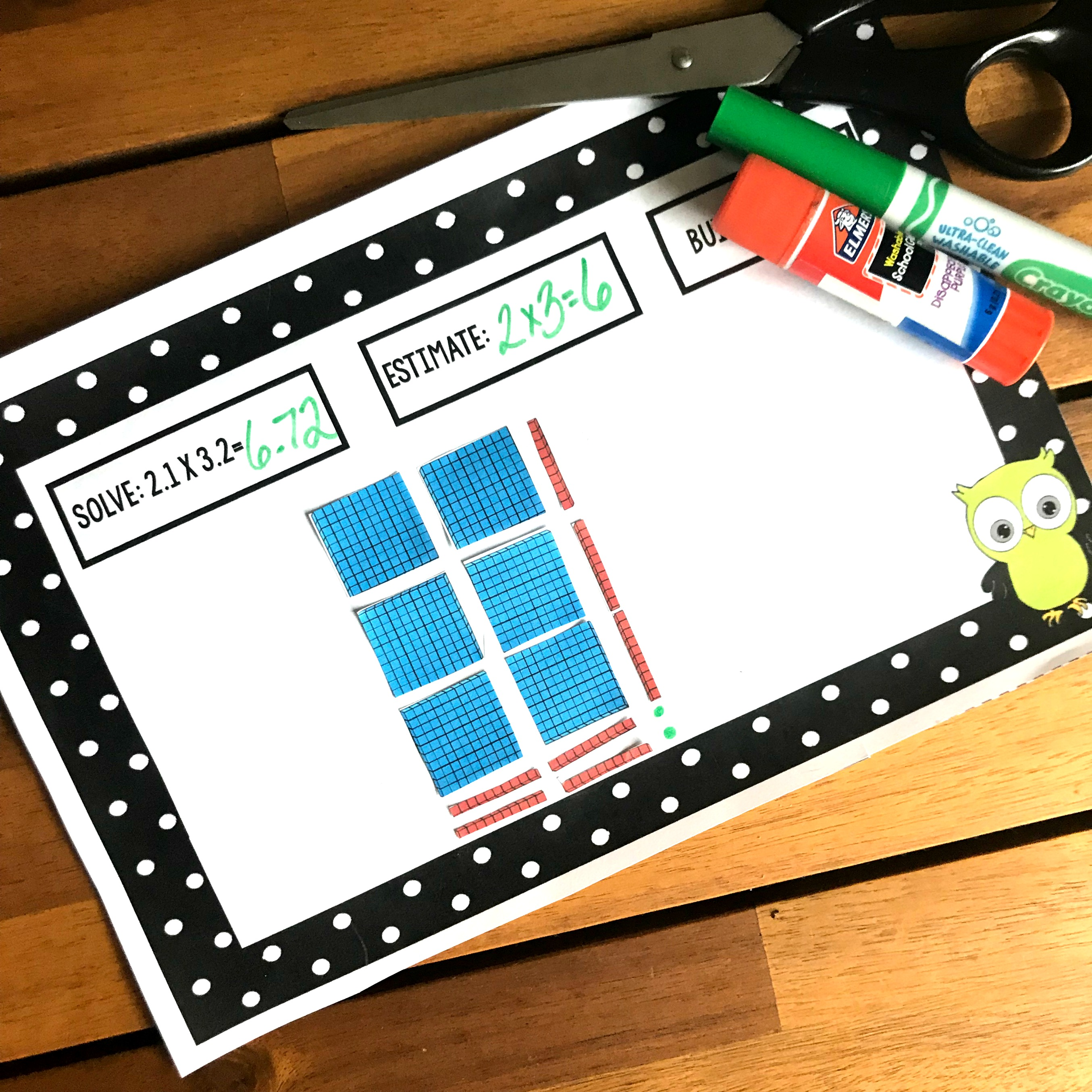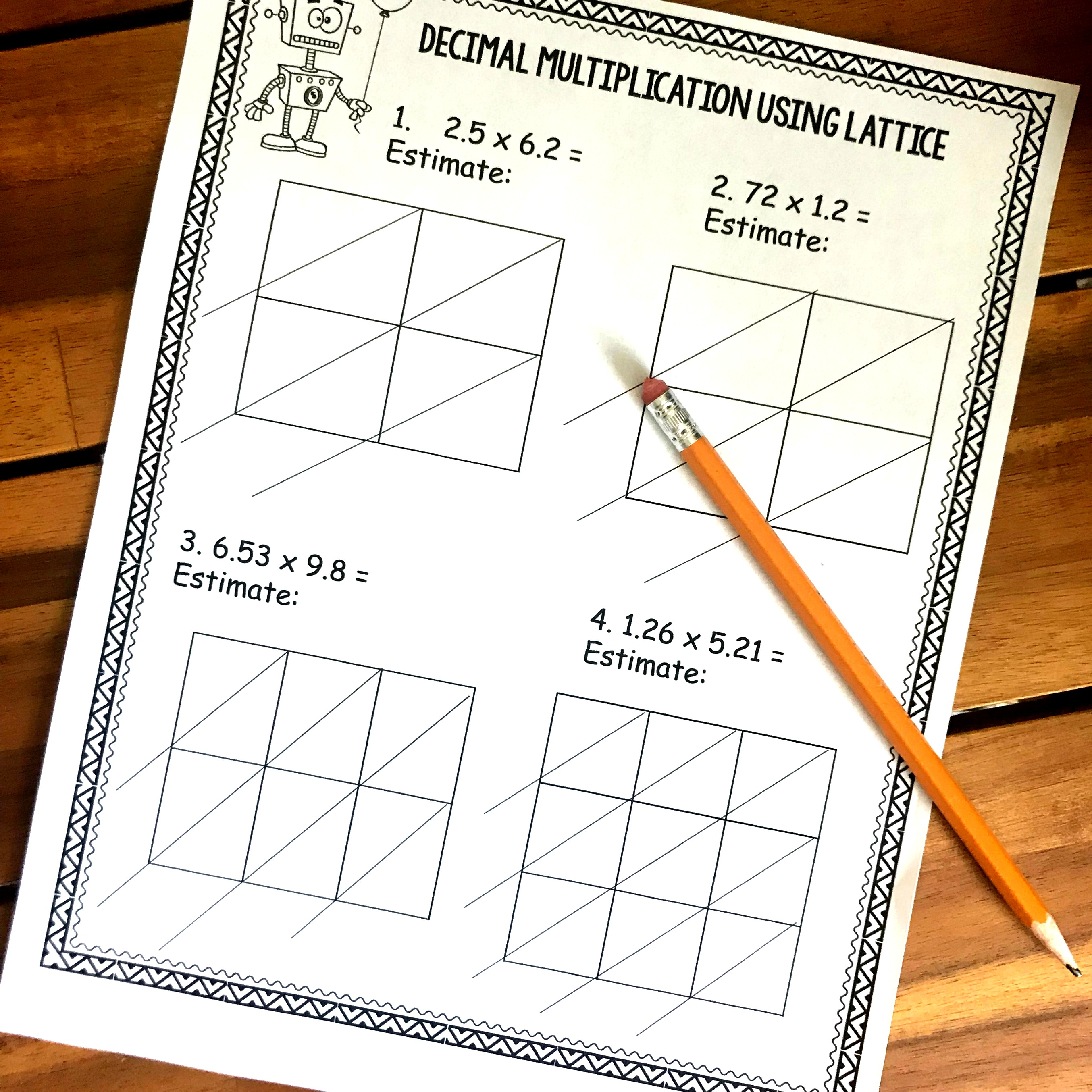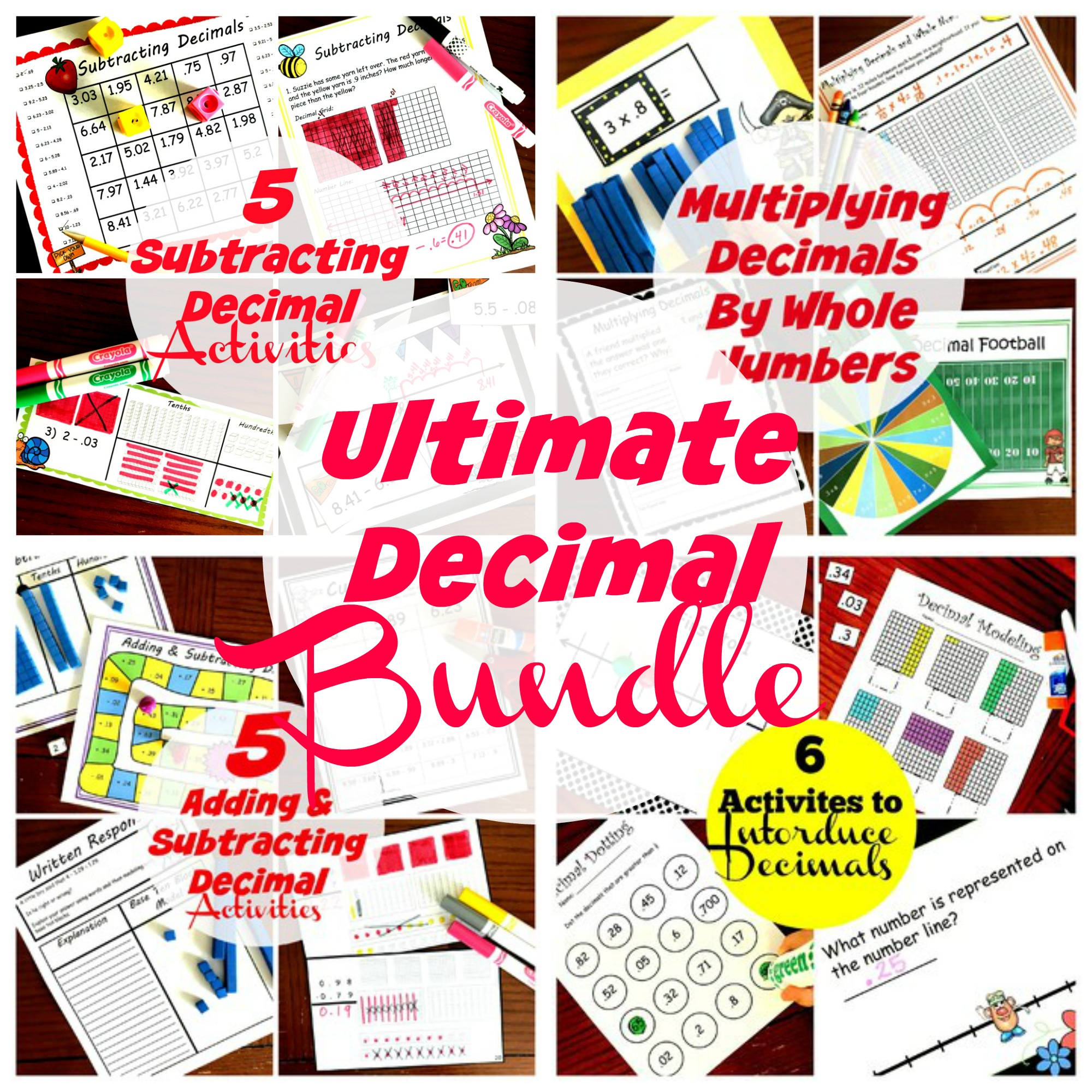Home » Math » FREE Interactive Notebook To Teach Lattice Method with Decimals

# FREE Interactive Notebook To Teach Lattice Method with Decimals

Want to teach the lattice method with decimals? This interactive notebook will take you through each step!! You may also want to check out my other interactive notebooks!!!

Interactive notebooks.

During the last two years as a public school teacher, my school worked on implementing interactive notebooks.

I loved them. They were fun, hands-on and gave children a record of what they had learned.

Another benefit I found, was that they could be used to walk children step by step through a math problem. And when you are teaching a method that isn’t traditional, that becomes helpful to the student as well as the parent!

Today, our focus turns to lattice multiplication and using it with decimals. It definitely isn’t traditional, but I found it worked well for many of my lower math students. So with further ado, here is a lattice method with decimals interactive notebook.

## Prep-Work

Some interactive notebooks require some prep-work, but this one doesn’t.

1. First, print off the pages.
2. Then provide pencils, highlighters, and glue.
3. Finally, have the children glue the notebooks into

## Lattice Method With Decimals

If you have used lattice to multiply large digit numbers, you know it is a matter of lining up numbers and multiplying single digit numbers.

The only difference here is that a decimal is added, and we have to figure out where the decimal goes.

### Step 1: Estimate

Whether a child is multiplying decimals in arrays, using the traditional method, or partial products……I always teach them to estimate first.

By doing this they know what the answer should be close to. When they place the decimal in the answer they can check themselves against the estimate and see if they are right.

In our example, we have 2.3 x .45.

We can round 2.3 down to 2 and .45 is close to 1/2. Now we find 1/2 of 2 which is 1.

So our answer should be around 1.

### Lattice Time

Now comes the fun part. Putting the decimal in the right place with the lattice grid.

Now detailed instructions are on the interactive notebook, but it is really very simple. All you have to do is this:

Place one finger on the top decimal. This finger will move down the horizontal line. Place another finger on the other decimal. This finger will move to the left. Move them till they meet. Then follow that diagonal line down, and this is where the decimal goes.

Our answer is 1.035, which is very close to our estimate! We did it.

I hope this lattice method with decimals helps your students to master multiplying decimals.

You’ve Got This,

Rachel

You may also like:

Multiplying Decimals With ArraysDecimal Lattice Practice